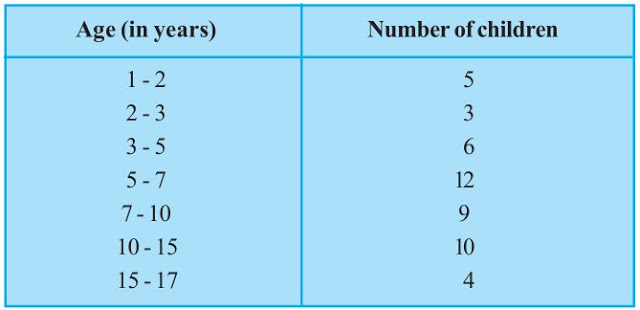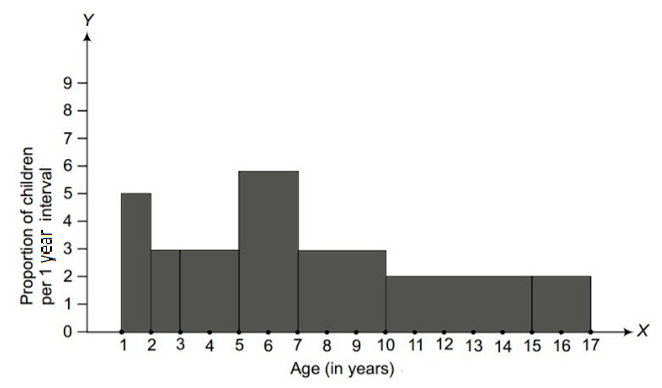# A random survey of the number of children of various age groups playing in a park was found as follows: Draw a histogram to represent the data above.Solution

The width of the class intervals in the given data is varying in nature. Also, The area of a rectangle is proportional to the frequencies in the histogram.

Thus, the proportion of the children per year can be calculated as given in the table below.

 Age (in years) Number of children (frequency) Width of class Length of rectangle 1-2 5 1 (5/1)×1 = 5 2-3 3 1 (3/1)×1 = 3 3-5 6 2 (6/2)×1 = 3 5-7 12 2 (12/2)×1 = 6 7-10 9 3 (9/3)×1 = 3 10-15 10 5 (10/5)×1 = 2 15-17 4 2 (4/2)×1 = 2

Let x-axis = the age of children

y-axis = proportion of children per 1 year interval